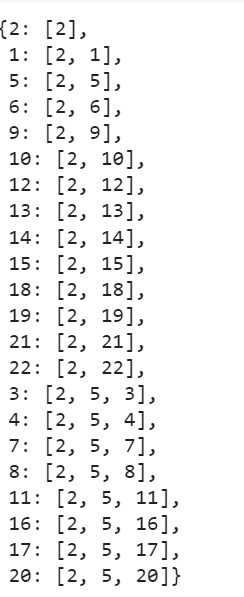# Shortest Path in Text Data, AI-Exercise from Research Perspective

Here is next article, which is written from research perspective. The aim of article is to form a base to cover shortest path problems in Textual Data. Graph based algorithms have a huge potential to be used in Natural Language Processing and in many Graph based problems. In this article I introduce you how, how to initialize Graph based analysis in Text file. Note, that Graph based algorithms are still in the arena of being used in research works for NLP and Text Mining.

Lets start with initializing of graphs from a Text Data. So let us make a Graph from Textual input which is a file as of now, means in this article.

Graph is a collection of vertices and edges connecting them along with the weights of these connection. This is a highly used data structure in computer science, and its use if often made in research in AI.

Step 1. We use networkx and initiliaze the graph G with it, sentences is the list of text fragments in the input.

`import networkx as nxG = nx.MultiDiGraph()sentences  = []inputMediumfile = open("/content/File1.txt")num_sent = 1for sentence in inputMediumfile:sentences.append(sentence)G.add_node(num_sent)num_sent = num_sent+1`

Step 2. Now adding edges to the Graph, if the similarity between the two vertices in the Graph just created is greater that a given threshold

`import matplotlib.pyplot as pltprint('Making of non complete graph from these nodes, avoiding self loops')def edge_Weight(i,j):########################similarity code as per requirement ###########return distance_ti=0j=1for i in range(1, num_sent-1):for j in range(1, num_sent-1):weight = edge_Weight(i,j)if  weight >= threshold:G.add_edge(i,j, weight+1)print("Vertex set: ",G.nodes())print("Edge set: ",G.edges())nx.draw(G, with_labels=True)plt.show()`

Step 3. Find the shortest path from node 2,

`nx.shortest_path(G, 2)`

Sample Output